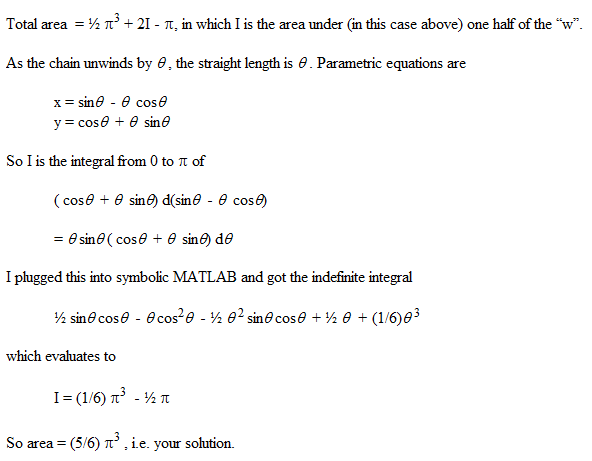# Problem 97 Solution

Lets call x the angle formed by (1,0), (0,0), and the point where dog's chain leaves the edge of the lighthouse, assuming the dog is trying it extending as far as possible causing the straight part of the chain to be tanjent to the edge of the lighthouse. For now lets only concern ourselves with the area to the right of x=-1 and above y=0.

Since the radius of the lighthouse is 1 the length of the straight part of the chain is the same as x. Through a little trigonomtry we can get the coordinates of the dog given x as (cos(x)+x*sin(x),sin(x)-x*cos(x)). The distance from (0,0) to the dog, given x, conveniently works out to (1+x2)1/2, using the length of a line segment formula.

Lets call y the angle fromed by (1,0), (0,0), and the location of the dog. Through some more trigonomty (again left up to you) we can solve for y in terms of x as y=x-tan-1. Now we are ready to integrate.

The area in terms of polar coordinates is 1/2 * the integral over the range of the angle of the radius squared. We can not simply take the integral from 0 to Π of 1+x2 because this is not the radius formed by the angle x, but the radius formed by y. We could take the integral over the range of the angle y but finding the coordinates of the dog in terms of y is very hard (I couldn't do it). However we can take the ingregal as x goes from 0 to Π of 1+x2 multiplied by the change in y given a change a change in x. Since y=x-tan-1(x). dy/dx = 1-1/(1+x2)!

So now the area becomes the integral as x goes from 0 to Π of 1/2 the product of 1+x2 and 1-1/(1+x2) which equals 1/2 the integral from 0 to Π of x2 which equals Π3/6.

This integral, however, does not cover the area bounded by the triangle (0,0), (-1,0) and (-1,Π). So we must add this area which is Π/2. Yet we must subtract the area inside the lighthouse which the dog may not go in, which is also Π/2. So these two modifications conventiently cancel each other out.

The area of the quarter circle to the left of x=-1 and above y=0 is Π3/4, since the length of the chain is Π and the dog covers a semicircle to the left of the lighthouse. So the total area above y=0 is 5/12 * Π3. Finally double this for the area under y=0 and the total area is 5/6 * Π3

Here is another solution I received from Patrick.I would like to thank Steven Lutz for sending me this problem.

Michael Shackleford, A.S.A.